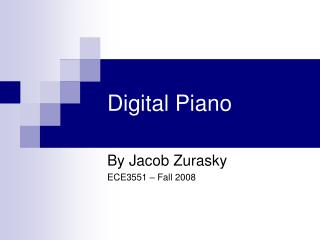DownloadDownload PresentationDigital Piano

# Digital Piano

Download Presentation## Digital Piano

- - - - - - - - - - - - - - - - - - - - - - - - - - - E N D - - - - - - - - - - - - - - - - - - - - - - - - - - -
##### Presentation Transcript

1. Digital Piano By Jacob Zurasky ECE3551 – Fall 2008

2. Project Goals • Implement many inputs • Synthesize a piano-like tone • Play tones based on inputs • Play a song from memory

3. Inputs • BF533 board only has 4 input switches • 32 inputs implemented via 8x4 matrix • PIC scans matrix, sends a packet of data • BF DMA channel stores data into array

4. Communications • RS232, 9.6 kbps data transmission • 6 bytes = 48 bits of data • Each bit represents a key on the piano • Bit is low when a key pressed

5. UART Configuration • UART_LCR - 8 data bits, 1 stop bit • UART_DLH/DLL - 0x16 = 352 • Baud rate = SCKL / (16 * Divisor) = 9588 kbps, with 0.1% error • UART_IER - Generate Rx Interrupt

6. UART DMA Channel • UART Rx Interrupt triggers a DMA write • DMA writes 6 elements to Rx_Buffer[] • Rx_Buffer[] holds the last packet sent

7. Tone Synthesis • Pure sine wave at a given frequency sounds very empty • Add richness to sound by adding harmonics • Analyze frequency spectrum of a piano sample

8. Pure Tone Spectrum Analysis

9. Piano Spectrum Analysis

10. Fourier Series • Use a fourier series to generate a signal with a similar frequency content • Add many harmonics of the fundamental frequency, at lower amplitudes • Composite signal has a richer sound

11. Fourier Series • y = a1 * sin[2 * Pi * ((1 * f) / fs) * t] + a2 * sin[2 * Pi * ((2 * f) / fs) * t] + a3 * sin[2 * Pi * ((3 * f) / fs) * t] + a4 * sin[2 * Pi * ((4 * f) / fs) * t] + … + an * sin[2 * Pi * ((n * f) / fs) * t]

12. Components of Output

13. Composite Output

14. Spectrum Analysis Comparison

15. Tone Synthesis • Fourier series has over 50 components • sinf() function too slow to call multiple times per sample • Implement a look-up table • Each octave of notes is stored to an array

16. C3 Octave Array

17. Tone Synthesis • Each note has three characteristics • Key_Prev_State - Previous state of note • Key_Cur_Period - Location within period • Key_Decay - Current decay rate of note • Each octave has an array of note lengths • Each octave has an array of note offsets

18. Tone Synthesis • D3, 3rd note in C3 octave • C3_Period = 327, C3_Offset = 714 • Notes3 is the first sample • Notes3 is the last sample

19. Tone Synthesis • Linear decay while note is held • Key_Decay and Key_Cur_Period are incremented after each sample if the note is active • Summation of look-ups allows multiple tones simultaneously

20. Playback • Events stored on PIC similar to MIDI • Each event has a two byte frame offset • 6 bytes of data for the key status